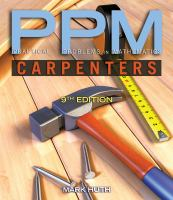Close

# Practical Problems in Mathematics for Carpenters

## by Huth, Mark• ISBN: 9781111313425
• ISBN10: 1111313423

# Practical Problems in Mathematics for Carpenters

## by Huth, Mark

• List Price: \$79.95
• Edition: 9
• Publisher: Cengage
• Publish date: 07/26/2011
• ISBN: 9781111313425
• ISBN10: 1111313423
used \$46.27
You save: 42%
new \$73.45
You save: 8%
FREE economy shipping!
ebook \$24.57
Description: Preface. Section I: WHOLE NUMBERS.1. Addition to Whole Numbers. 2. Subtraction of Whole Numbers. 3. Multiplication of Whole Numbers. 4. Division of Whole Numbers. 5. Combined Operations with Whole Numbers. Section II: COMMON FRACTIONS.6. Addition of Common Fractions. 7. Subtraction of Common Fractions. 8. Multiplication of Common Fractions. 9. Division of Common Fractions. 10. Combined Operations with Common Fractions. Section III: DECIMAL FRACTIONS.11. Addition of Decimal Fractions. 12. Subtraction of Decimal Fractions. 13. Multiplication of Decimal Fractions. 14. Division of Decimal Fractions. 15. Expressing Common Fractions and Mixed Numbers as Decimals. Section IV: PERCENT AND PERCENTAGE.16. Simple Percent and Percentage. 17. Interest. 18. Discounts. Section V: MEASUREMENT: DIRECT AND COMPUTED.19. Linear Measure. 20. Working with Feet and Inches. 21. Square Measure. 22. Surface measurement: Triangles. 23. The Framing Square. 24. Surface Measurement: Irregular Figures. 25. Surface Measurement: Circles 26. Volume Measurement: Cubes and Rectangular Solids. 27. Board Measure. 28. Volume Measurement. 29. Weight Measure. Section VI: POWERS AND ROOTS. 30. Applications of Exponents in Formulas. 31. Using Square Root to Find Sides of Right Triangles.
Expand description